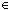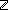IF YOU CAN SEE THIS go to /sfa/Nuprl/Shared/Xindentation_hack_doc.html

### Executing Expressions

Terms in Nuprl provide the syntactic entities used for expressions. In Nuprl, one might say, terms are adopted as expressions for mathematical objects when Types are defined. The terms become notations for the values of that type, such as 1+2, and sometimes notations for the types themselves, such as "" itself.

We follow a method given by Per Martin-Lof.

Prior to, that is independently of, using terms to denote mathematical values in this way, one defines the raw, untyped computational behavior of terms. This behavior is then used in part to explain how mathematical values of various types shall be denoted.

All that the semantics of types requires is that we know how to execute, or "evaluate", closed terms. (A closed term is one with no free variables.) If such execution produces a result, that result is sometimes called the "value" of the term; beware that, in this sense, the value of "1+2" is not the number 3, but is instead a term "3", i.e., a numeral.

All closed terms are classified as having "canonical" or "non-canonical" form, and whether a term is in canonical form depends only on its outermost form. Specifically, whether a term is in canonical form is preserved by any change of binding variables or subterms.

Any closed term in canonical form simply evaluates to itself. Any result of evaluating a term must be closed and have canonical form. A key part of introducing a new primitive operator into the semantics is to explain how to evaluate it, and this explanation must be independent of type definition. A term can have at most one value.

Here is an example.

The pair constructor <a,b> is in canonical form.
A closed expression of form w/x,ye(x;y) has a value R iff w has some value <a,b> and e(a;b) has value R. See Pairs.

And another.

The left- and right-injectors inl(a) and inr(b) are in canonical form.
A closed expression of form InjCase(wxe(x); yf(y)) has a value R iff either w has some value inl(a) and e(a) has value R or else w has some value inr(b) and f(b) has value R. See Disjoint Union.

See Canonical Forms and NonCanonical Forms for lists and descriptions of various primitive operators.

(Dec 2001 - sfa )